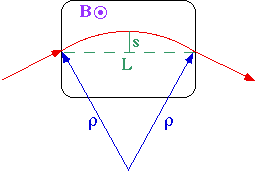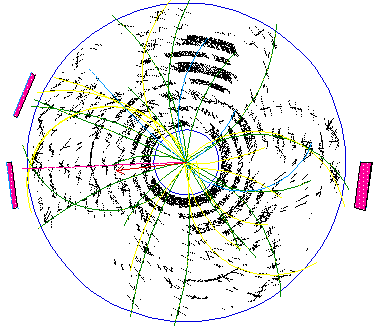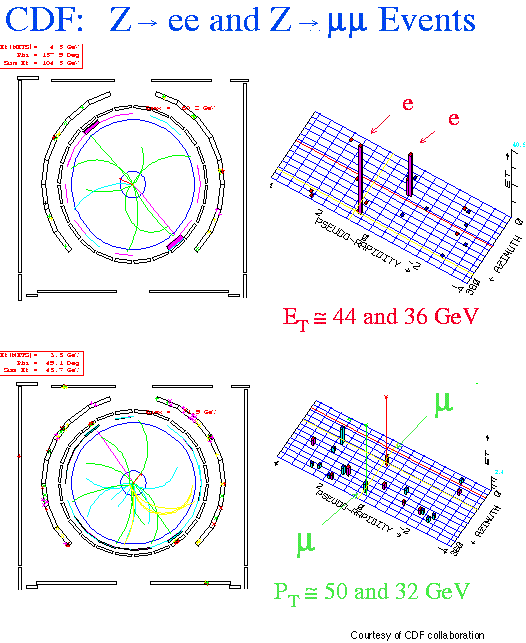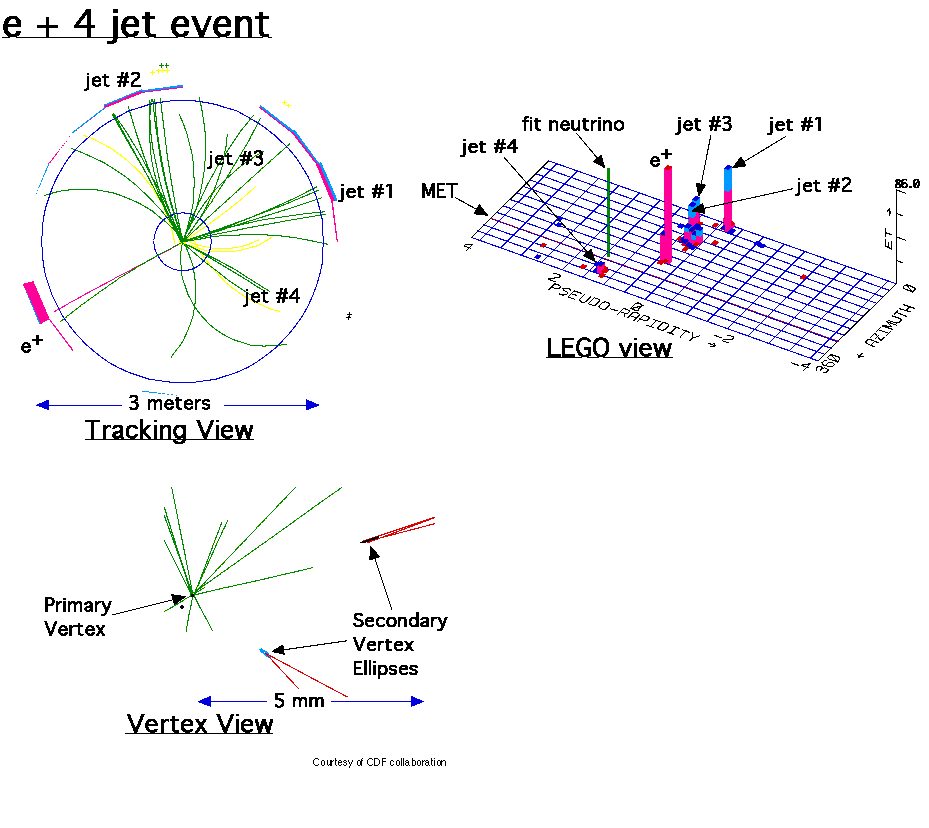Some Detector Requirements

Typical high energy physics example

proton-antiproton Þ Z0+X
Z0 Þ e+e-

To observe Z0, need to measure e+e- and reconstruct mass:

M2(e+e-) = E2-p2 = 2(me2 + Ee+Ee- - Pe+·Pe-)
= 4Ee+Ee-sin2
q/2   (for Ee+,Ee->>me)

where q is the angle between the e+ and the e-. For high energy symmetric decays,

M ~ E·q   (for Ee+,Ee->>me)

("E" is now the typical electron/positron energy) and

(sM/M)2 ~ (sE/E)2 + (sq/q)2

We'd like the experimental uncertainty to be less than the natural width:

GFWHM(Z0) = 2.7 GeV   Þ   sZ/MZ ~ 1%

Typically E~M, and the tracking detector radius is about 1m, so q~1 and

sq/q < 1%    Þ   sx < 1cm

The best collider calorimeter in the world (ZEUS) has systematic uncertainties of 1-2%. If the electron energy is determined by tracking detectors, then we need sP/P~1%.

Measuring momentumBy simple geometry, the radius of curvature is

r = L2/(8s) + s/2

So for a high energy (s<<L) unit charge (q=e) particle

P = 0.3 B L2/(8s)    (Units: GeV, m, T)

If B & L are well known, then

\ sP/P = ss/s = 8 ss P/(0.3 B L2)

Typical high energy experiments (CDF & ZEUS) have B~1.5T and tracking detector radius of about 11/2m, so

\ sP/P ~ 1% at P~102 GeV    Þ   ss/s ~ 0.01mm!

For N~102 measurements still need at least ss/s ~ 0.1mm.

It is not for nothing that 4 Nobel prizes have been given for tracking chambers:

Wilson - cloud chamber

Powell - photographic emulsion method

Glaser - bubble chamber

Charpak - wire chamber

Production of an W- baryon in the CERN 2m bubble chamber.CERN PHOTO

(Bubble chamber pictures are studied in the High Energy Physics experiment in the undergraduate labs.)

Drift Chambers:The time of arrival of the ionization electrons (relative to a start from a scintillator) is measured and the drift distance determined from the drift velocity. Drift chambers have thousands of wires, e.g. CDF or ZEUS.Courtesy of CDF Collaboration

Antiproton-proton collisions at a c.m. energy of 1.8 TeVCourtesy of CDF Collaboration

CDF Central Detector: The hadronic calorimeters detect hadrons by causing them to collide many times with iron plates. In these collisions, other particles are produced. These particles cross sheets of scintillating plastic where they give off small flashes of light. A measurement of the total amount of light measures the energy of the hadron.

CDF Forward Detectors : There are also two forward detectors; so named because they are arranged along the main accelerator beam in front of and in back of the central detector. In the linked photograph, one can see these detectors in the assembly hall area outside the main machine before they were installed.Example Z events: Two events are shown, one Z --> two electrons and one Z --> two muons. Each event has a tracking view showing the charged tracks in the bend plane (we call this the r-phi view for radius-azimuth) of the the 1.4 Tesla CDF solenoid magnet. The diameter of the circle is about 3 meters. The "lego" plot is also shown for each event -- with "stacks" of electromagnetic (red) and hadronic (blue) energy seen in the calorimeter towers. Think of this "lego" plot as the surface of a cylinder around the solenoid unwrapped into a plane. Note in the Z--> two muon event the calorimeter energy scale is very small since the muons penetrate the calorimeter and leave only "minimum ionizing" pulse height. So we identify the muons from their charged tracks and hits in the Muon Chambers represented by the square box drawn outside of the 3 meter tracking circle. This box is not drawn to scale -- in the experiment the box is about 10 meters by 10 meters. Look closely to see the "hits", drawn as x's in line with nearly straight (therefore high momentum) tracks in the circle. In the lego plot we have drawn vertical lines to represent the energy of the muon tracks. Note the difference in the Z --> e+e- event where there are straight tracks pointing at clumps of red (electromagnetic) energy and no hits in the muon chambers."GOLDEN" Top Event - This event has a 109 GeV e+, and 4 Jets of (1) 90, (2) 77, (3) 64, and (4) 38 GeV. Jets (1) & (4)are tagged as b-jets by the CDF Silicon Vertex Detector. Jets (1,2,3) are from top; (2) & (3) are from a W. The event fit gives a Top Quark Mass of 170 ± 10 GeV.